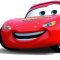## Number of pairs with maximum sum in C++Published on 03-Jul-2021 08:33:33
Given an array, we need to find the number of pairs with maximum sum. Let's see an example.Inputarr = [3, 6, 5, 2, 1, 2, 3, 4, 1, 5]Output2 The maximum pair sum is 10. There are 2 pairs with maximum sum. They are (5, 5) and (6, 4).AlgorithmInitialise the ... Read More

## Number of pairs with Bitwise OR as Odd number in C++Published on 03-Jul-2021 08:31:52
Given an array, we have to find the number of pairs whose Bitwise OR is an odd number. Let's see the example.Inputarr = [1, 2]Output1 There is only one pair whose Bitwise OR is an odd number. And the pair is (1, 2).AlgorithmInitialise the array with random numbers.Initialise the count ... Read More

## Number of pairs whose sum is a power of 2 in C++Published on 03-Jul-2021 08:29:53
Given an array, we have to find the number of pairs whose sum is a power of 2. Let's see the example.Inputarr = [1, 2, 3]Output1 There is only one pair whose sum is a power of 2. And the pair is (1, 3).AlgorithmInitialise array with random numbers.Initialise the count ... Read More

## Number of pairs from the first N natural numbers whose sum is divisible by K in C++Published on 03-Jul-2021 08:26:28
Given numbers N and K, we have to count the number of pairs whose sum is divisible by K. Let's see an example.InputN = 3 K = 2Output1 There is only one pair whose sum is divisible by K. And the pair is (1, 3).AlgorithmInitialise the N and K.Generate the ... Read More

## Number of ordered points pair satisfying line equation in C++Published on 03-Jul-2021 08:24:00
The line equation that should be satisfied is y = mx + c. Given an array, m, and c, we have to find the number of order points satisfying the line equation. Let's see an example.Inputarr = [1, 2, 3] m = 1 c = 1Output2The pairs satisfying the line ... Read More

## Number of non-negative integral solutions of sum equation in C++Published on 03-Jul-2021 08:21:10
In this tutorial, we are going to write a program that finds the number non-negative integral solution of sum equation.The sum equation is x + y + z = n. You are given the number n, you need to find the number of solutions for the equation. Let's see an ... Read More

## Number of nodes greater than a given value in n-ary tree in C++Published on 03-Jul-2021 08:19:18
Given an n-ary tree and a number, we have to count the number of nodes greater than the given number. Let's see an example.Inputtree = [, [1, 2], [3, 5]] n = 2Output3There are 3 nodes with values that are greater than n.AlgorithmInitialise the n-ary tree.Initialise the count to 0.Increment ... Read More

## Number of NGEs to the right in C++Published on 03-Jul-2021 08:16:54
You are given an array and the index of the target element. We have to count the number of elements greater than the given element to its right. Let's see an example.Inputarr = [2, 3, 5, 1, 4, 2, 6] index = 3Output3The target index element is 1. There are ... Read More

## Number of n digit stepping numbers in C++Published on 03-Jul-2021 08:15:20
The stepping number is a number where the difference between the consecutive digits is 1. You are given a number n representing the number of digits. You need to count the total number of stepping numbers with n digits.Let's see an example.Input2Output17The lowest number of 2 digits is 10 and ... Read More

## Number of moves required to guess a permutation in C++Published on 03-Jul-2021 08:13:03
Given a number N, we need to find the moves required to guess the permutation in the worst-case scenario. The number of moves required to guess the permutation will be n!. Let's take an example.Input5Output129When we have 5 elements, then we have 5 ways to guess, and 4 ways when ... Read More